~ click REFRESH to restart animation ~

# PTOP: Periodic Table Of PRIMES: How to Make with Fractals

## Introduction

What if the secret of the primes were to be more than just atomic-base units of the natural whole numbers.

What if they really gained their constructive prowess when their natural sequence — the PRIMES Sequence (PS = 3,5,7,11,13,17,19,23,29,31,...) — were tied together as PSs.

Yes as FRACTALS! PS-Fractals.

(Side Note: This is easy enough to do, especially if we disregard PRIME 2, as ALL the remaining PRIMES are ODD #s. PRIME 2, the ONLY EVEN PRIME, could be thought of as composed of PRIME 1 + PRIME 1, but since we now do not allow 1 to be PRIME, 2 being there is really quite silly — a techno-derived result. Lose the 1, lose the 2!)

The PS-Fractals are characterized as:

1. The strictly follow the PS;
2. The spacing — PRIME Gaps — between the PS is strictly followed;
3. When combined with another PRIME in sets — P1, P2 — to form PPsets, only 1 member of the set is variable, the other remains constant, as the sets form groupings, strings or “TRAILS” of connected PPsets.

These three simple rules, combined with the PS-Fractal Set will combine —concatenate — with another PS-Fractal Set at each subsequent PS step within a given sequence and will allow one to form the entire PTOP (Periodic Table Of PRIMES) from scratch, without any direct reference to the BIM (BBS-ISL Matrix) from which it was originally derived.

Furthermore, and not without enormous significance, the P1, P2 members of ANY and ALL PPsets that sum to equal an EVEN # (Goldbach Conjecture), are ALWAYS symmetrically spaced (STEPS) from either side of the said EVEN/2.

This can be seen on the PTOP by simply dividing the EVEN by 2 and observing that the PPset members are always equal STEPS away to either side, except when the TRAIL ends and P1 = P2.

On the BIM, dividing the EVEN by 2 leads to a straight-line path to EVEN/2 on the Axis, and there one sees a very clear visual of how PPset members are always equal STEPS away to either side, except when the TRAIL ends and P1 = P2. Additionally, lines drawn from each respective P1, P2 will meet on that straight-line path at 90° forming a Right-angled isosceles triangle. All such PPsets that compose any said EVEN will have their Right-triangles on this same line.

The geometry of the PS-Fractals is clearly shown on the BIM and one could easily say is an important and inherent part of the very same Inverse Square Law (ISL) that informs the spacetime structure of the Universe!

See the PRIMES Index

Outline:

1. PTOP derived from the BIM;
2. How to Make the PTOP from the BIM;
3. Simple, fractal-based way to directly make the PTOP.

## The PTOP is derived from the BIM (steps 1-10):

Proof of the Goldbach Conjecture (strong form, ≥6) (See images below text)

1. Natural (n), Whole Integer Numbers (WIN) — 0,1,2,3,…infinity — form horizontal and vertical Axis of a simple matrix grid.

2. The squares of such WINs — n2=12=1, 22=4, 32=9,…infinity — forms the central Diagonal of said grid — dividing it into two bilaterally symmetric triangular halves.

3. Every Inner Grid (IG) cell within is simply the difference (∆) between its horizontal and vertical Diagonal intercept values. They extend to infinity. The Diagonal WINs form the base of a 90° R-angled isosceles triangle with said IG cell value at the apex.

4. Every IG cell within is also the product of two Axis WINs (Either horizontal or vertical, not both), that form the base of a 90° R-angled isosceles triangle with said IG cell value at the apex.

5. The complete matrix grid extends to infinity and is referred to as the BIM (BBS-ISL Matrix).The BIM forms — and informs — a ubiquitous map (algebraic geometry) to:

• ​ The Inverse Square Law (ISL);

• The Pythagorean Triples (PT);
• The PRIMES (stealthily hidden, but revealed by NPS (Number Pattern Sequence).
6. The 1st Diagonal that runs parallel to either side of the main Prime Diagonal (PD, not of PRIME numbers, but primary), is composed of the ODD WINs: 1,3,5,…infinity.

7. If we add +1 to each value, that 1st Diagonal now becomes a sequence of ALL the EVEN WINs (≥4): 4, 6, 8,…infinity. NOTE: this is why the PTOP is hidden, in the normal, base BIM these remain ODDs.

8. Select ANY EVEN WIN and plot a line straight back to its Axis WIN — that Axis WIN = EVEN/2 = core Axis #.

9. Upon that same Axis, PRIME Pair sets (PPsets) — whose sum (∑) equals the EVEN WIN (on the 1st Diagonal) — will be found that form the base of 90° R-angled isosceles triangle(s) whose apex lie(s) on that straight line between the EVEN and its 1/2 Axis WINs. PPsets with identical PRIMES = 1/2 Axis value.

10. The proof that every EVEN WIN has ≥1 PPsets can be seen in the Periodic Table Of PRIMES (PTOP) that stealthily informs the BIM of how each and every EVEN WIN is geometrically related to one or more PPsets.

## How to Make the PTOP from the BIM (steps 1-10):

1. These PPsets are NOT randomly contributing their ∑s to equal the EVEN WINs, rather they come about as the consequence of a strict NPS: the sequential — combining, linking, concatenation — addition of the PRIMES Sequence (PS) — 3,5,7,11,13,17,19,…—to a base PS — 3,5,7,11,13,17,19,….

2. The NPS of this addition forms the PTOP: for each vertical PS — the 1st PRIME (P1) remains constant (3), the 2nd PRIME (P2) sequentially advances one (1) PS WIN — is matched diagonally with the 2nd PS, but now the 1st PRIME sequentially advances, while the 2nd PRIME remains constant within a given PPset.

3. This matching addition of the 2nd PS at the bifurcation point of the common 2nd PRIMES, forms the zig-zag diagonal PPset Trails that are the hallmark of the PTOP.

4. For every subsequent vertical PPset match, the Trail increases by one (1) PPset.

5. The rate of such PPset Trail growth far exceeds the PRIME Gap rate.

6. The zig-zag diagonal PPset Trails combine horizontally on the PTOP to give the ∑# of PPsets whose ∑s = The EVEN WIN.

7. More than simply proving the Goldbach Conjecture, the PTOP hidden within the BIM reveals a new NPS connection of the PRIMES: PRIMES + PRIMES = 90° R-angle isosceles triangles.

8. The entire BIM, including the ISL—Pythagorean Triples—and, PRIMES, is based on 90° R-triangles!

Similar to how the grid cell values of the Axis, PD and IG of the Pythagorean Triples reveal additional, intimate connections within the BIM, so too do the PPsets: the 1st PRIME values of each set points to the # of STEPS from the PD that intersects the given EVEN WIN (Axis2), on a straight line path back to its Axis, at the apex where its other PPset member intersects — this is no mere coincidence — and that apex is, of course, the 90° R-angle isosceles triangle that results. For example: EVEN = 24, Axis = 12, PD = 144, # of STEPS from PD towards Axis = 5 and 19 for the 5+19 PPset and 7 and 17 for the 7+17 PPset, and 11 and 13 for the 11+13 PPset that each forms the EVEN 24.

9. On the PTOP, the bifurcation concatenation of the PS — 3,5,7,11,13,17,19,..— forming the zig-zag diagonal Trail with the same vertical (Column) base PS — 3,5,7,11,13,17,19,…— of those respective EVEN WINs— that when “3” is subtracted, the remainder is a next-in-the-sequence PRIME — providing the remainder is itself NOT ÷ by 3, 5, 7, or any othe prime. Ex: 24 - 3 = 21, but 21 is ÷3, so no PS on the 24 EVEN Row. Ex: 22 - 3 = 19 and 19 is the PRIME within the PS located on that 22 EVEN Row.

10. Another set of STEPS (S) from the core Axis value directly on the BIM Axis, identifies each symmetrical pair of a given PPset that forms that given EVEN. These STEPS may by Universally calculated from the EVENS, P1 and P2 values. They follow a NPS on the PTOP. (See images below text).

## The Simple, Fractal-based method for making the PTOP (steps 1-5):

1. Place the PS along the Columns Header of your table (3,5,7,11,13,17,19,…53), ending with the EVENS #s header. If you like, you can also add the Sum (∑) PP #s header: the # of PPsets that make that EVEN.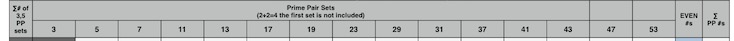2. Make a header Column on the far left side of the table for the ROWS Header, labeled ∑# of 3,5 PP sets and fill it in vertically down that Column with the natural number sequence (1,2,3,..) but leaving BLANK any Gaps in the PS: thus 1,2,3, BLANK Gap (for 9), then 4,5, BLANK Gap (for 15), then 6,7, BLANK Gap (for 21), then 23, BLANK Gaps (for 25,27), then 29,31, BLANK Gaps (for 33,35), then 37,… These #s reveal the length of the PPset Trails. The BLANK Gaps 9,15,21,.. are for the NON-Prime #s place holders.3. Now, for the really easy parts: Under Column Header 3 — and matching the entries in the Vertical Row Headers you just made above — enter 3+P2, where P2 is the PS (3,5,7,11,13,17,19,…). Here the PS is a fractal presented in a vertical array, with the P1 value being a constant 3.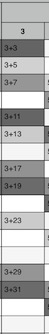4. The SAME PS fractal above is now going to form the rest of the table! Only now, its array will be skewed, forming a zig-zag diagonal. This diagonal will exactly follow the PS, only it will be skewed such that diagonal corner of PS 3 will go over 1 step and down 1 step to locate PS 5. PS 5 will go over 1 step, down 1 step to locate PS 7. Now, because there is a Gap of one (ODD#) between PS 7 and PS 11, one must from PS 7 go over 1 step, and down 2 steps to locate PS 11. This is the fractal-based NPS of the PS and once you get the pattern down, it is simple repeated identically starting at each PPset in the Rows under the Column Header 3. The only caveat is that the PPset Trail so formed truncates when P1 = P2.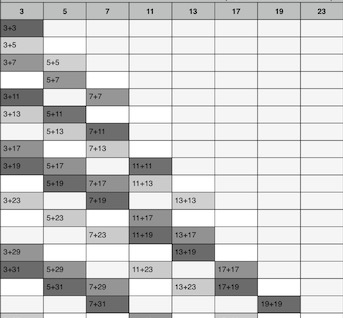5. Is quite helpful, but not required, to give some form of repeating graphic background tone to more clearly designate each PPset Trail, e.i. dark-light-med-dark-light-med.

Remember: every Trail is made of exactly the SAME Fractal Pattern: steps down from the 1st PPset (Column 1) —↓1—↓1—↓ 2—↓1— ↓2— ↓1—↓2—↓3—↓1...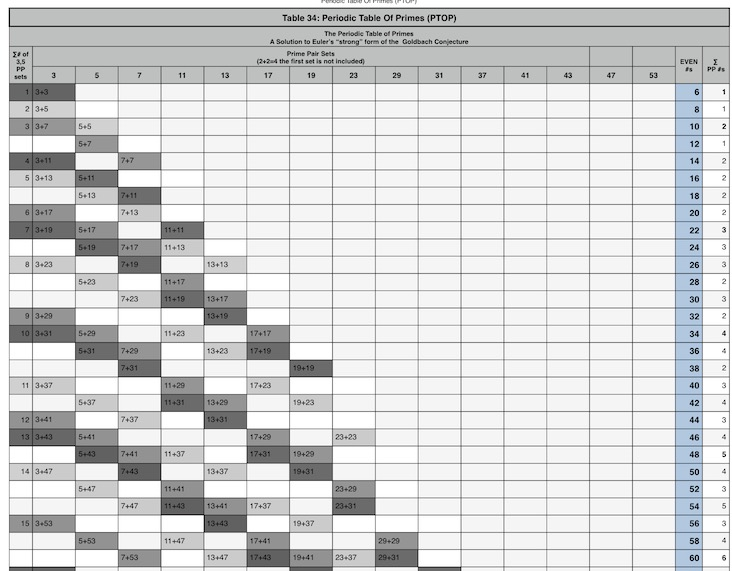## Images, Animations and Videos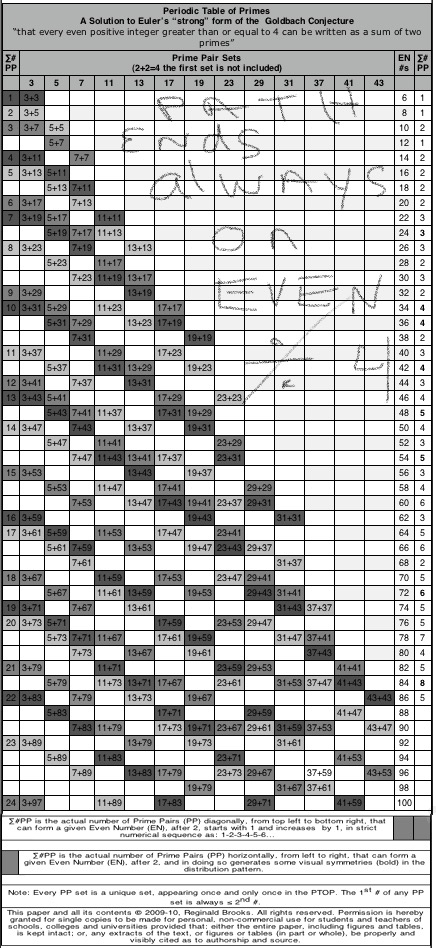Path ALWAYS ends on EVEN NOT ÷ 4.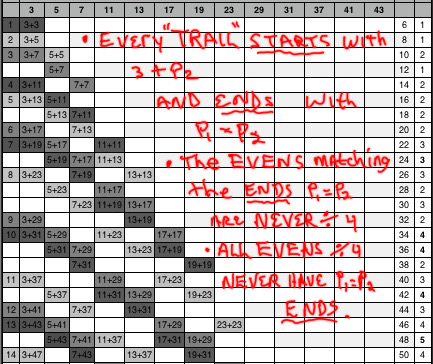Start and End of TRAIL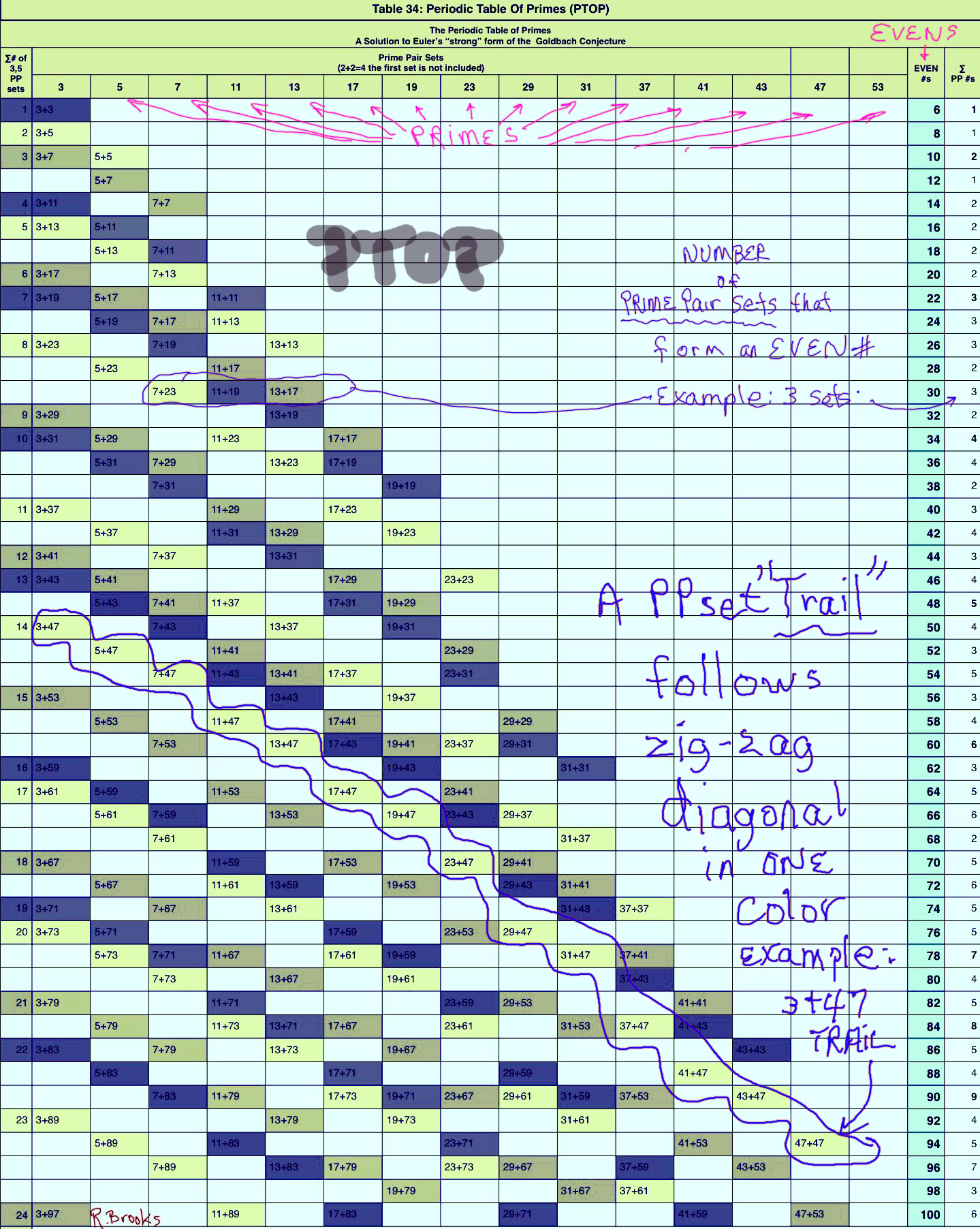PPset Trail follows a zig-zag diagonal down the PTOP.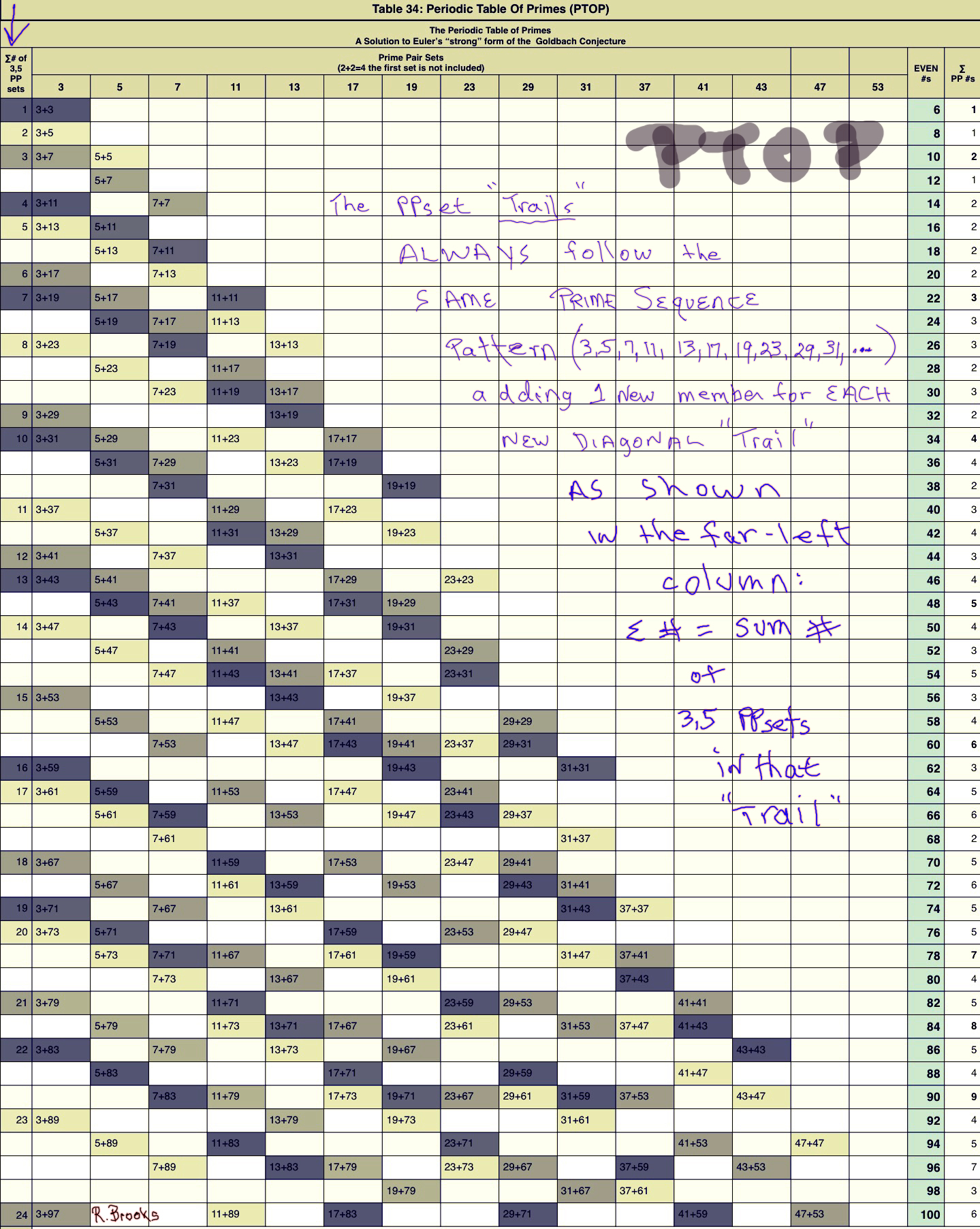The PPset TRAILS ALWAYS follow the same PS pattern, adding 1 new set for EACH vertical PS PPset.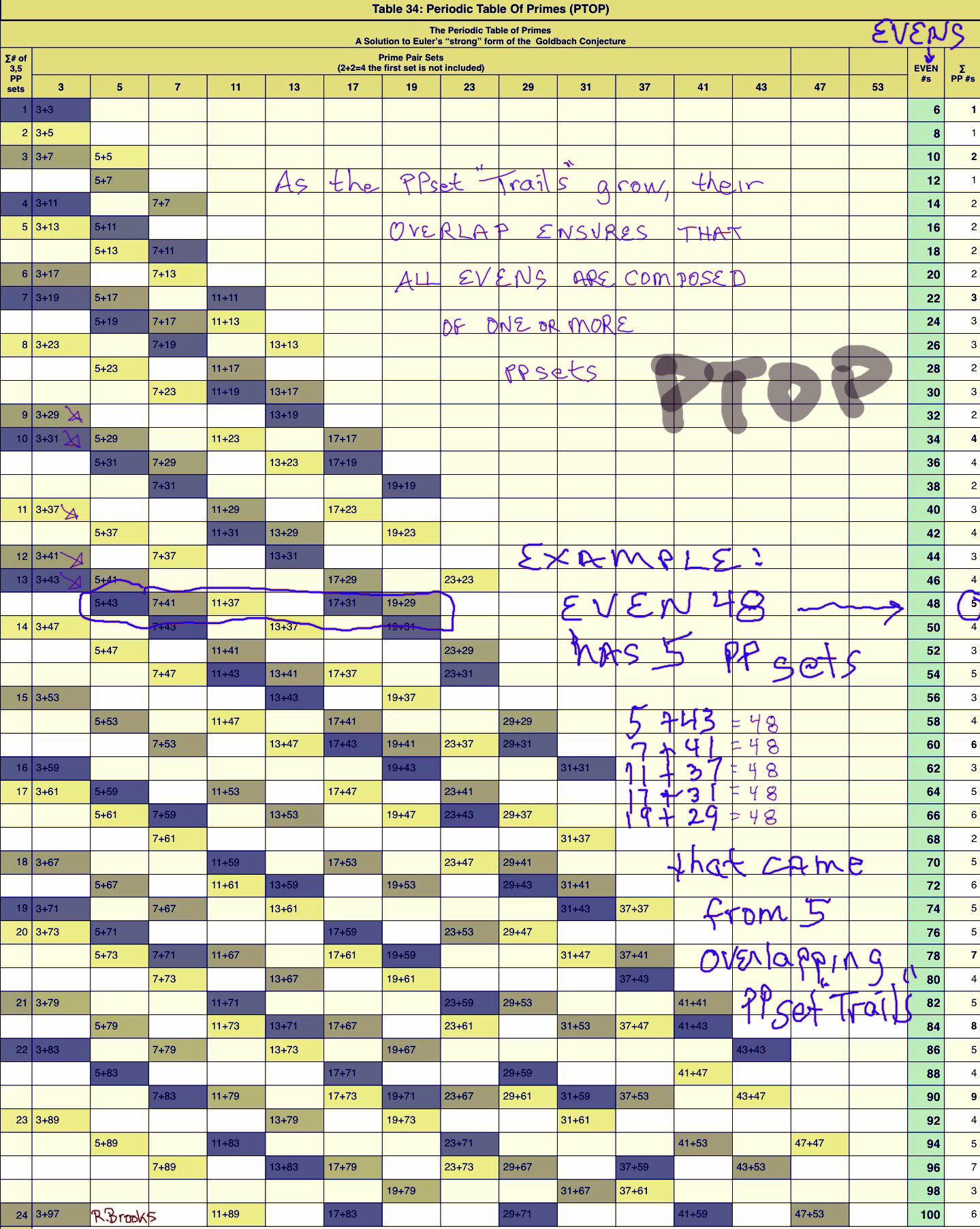As the PPset TRAILS grow, their overlap ensures that ALL EVENS are composed of the sums of one or more PPsets.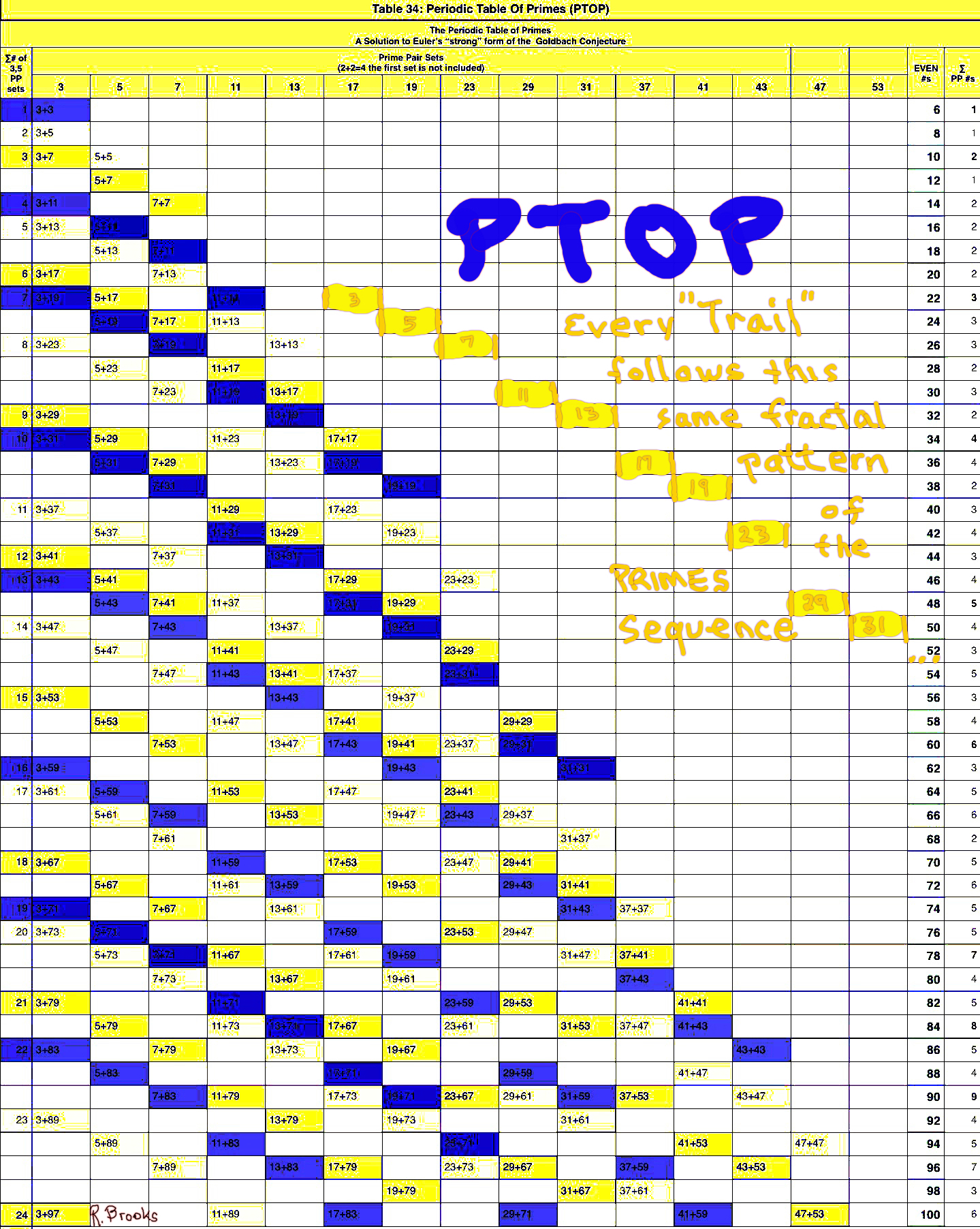Every PPset TRAIL follows the exact same PS-Fractal pattern.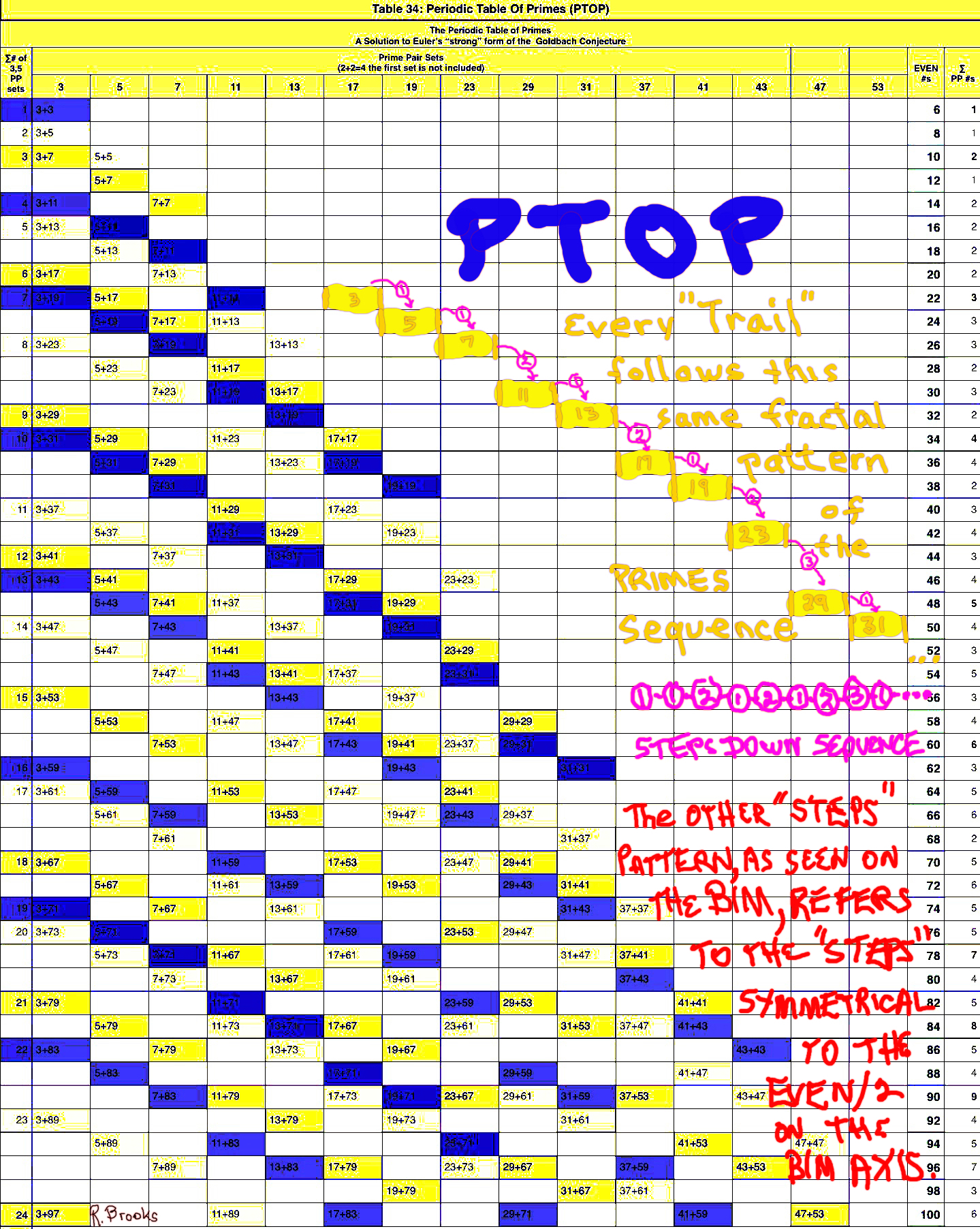Every PPset TRAIL follows the exact same PS-Fractal pattern: the steps – gaps – between subsequent PRIMES is fixed as 1-1-2-1-2-1-2-3-1...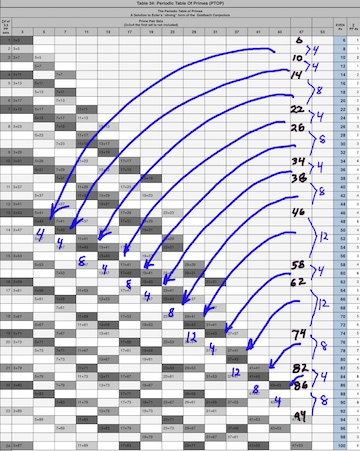Here we see that the differences (∆) between the EVENS that correspond to the END of the PPset TRAILS (P1 = P2). Dividing the ∆/4 = PS steps pattern.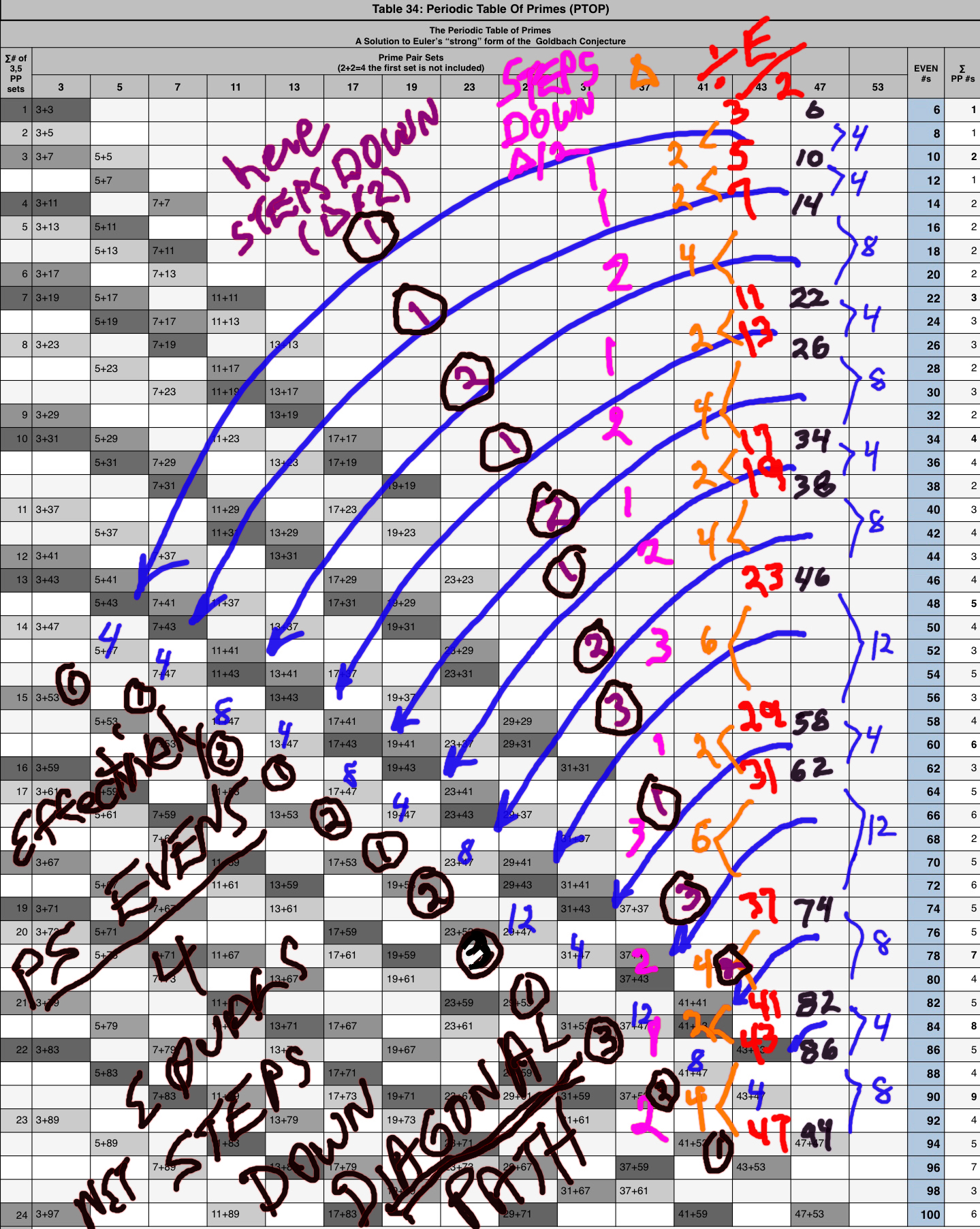Or we can simply take the differences (∆) between the EVENS at the TRAIL Ends directly and ÷ by 2 = PS steps sequence: 1-1-2-1-2-1-2-3-1… Effectively, the PS EVENS/4 = net steps down the Diagonal TRAIL.The RULE is that the PS EVENS are NEVER ÷ 4, while the NON-PS EVENS may or may not be ÷4, and those that are, are NEVER at P1 = P2 TRAIL Ends.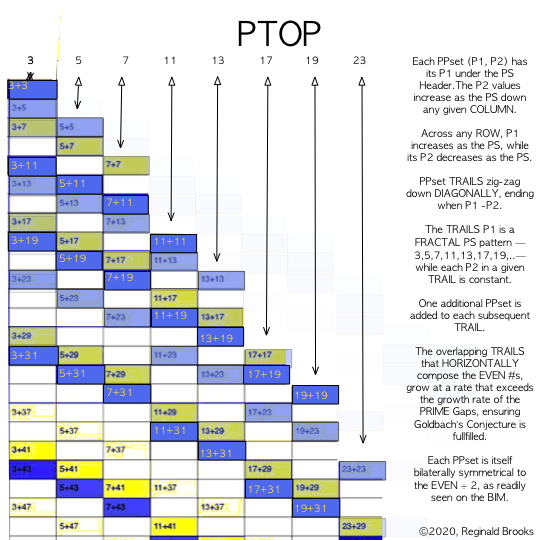Summary I.Summary II.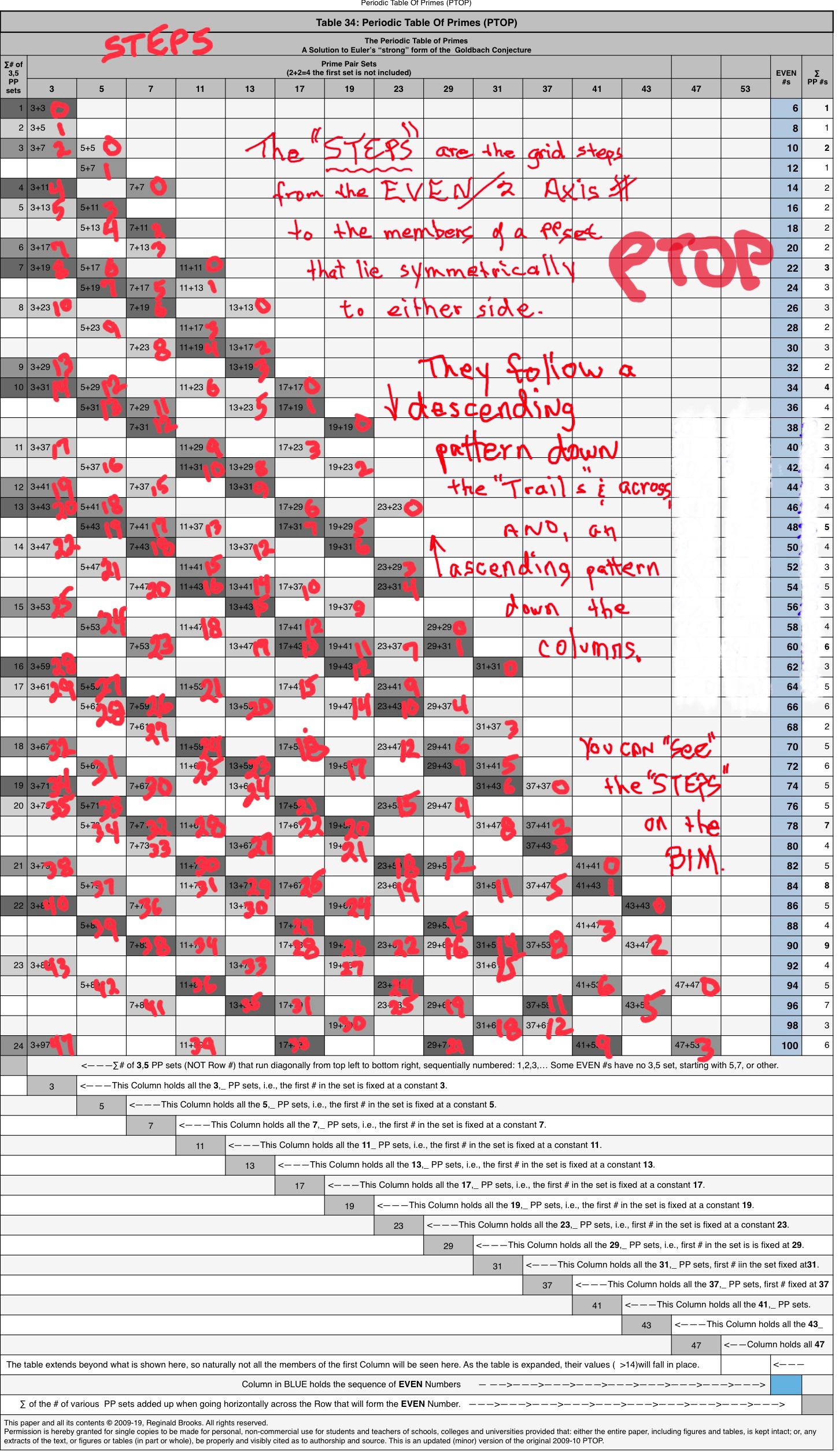The "STEPS" – not PS "steps" between the PRIMES – are the grid STEPS from the EVEN/2 Axis # on the BIM to the respective members of a PPset that lie symmetrically to either side. They follow a descending pattern down and across the TRAILS, and, an ascending pattern up the Columns. The PTOP is best for the summary pattern, while looking directly at the BIM is best for seeing the individual pattern(s).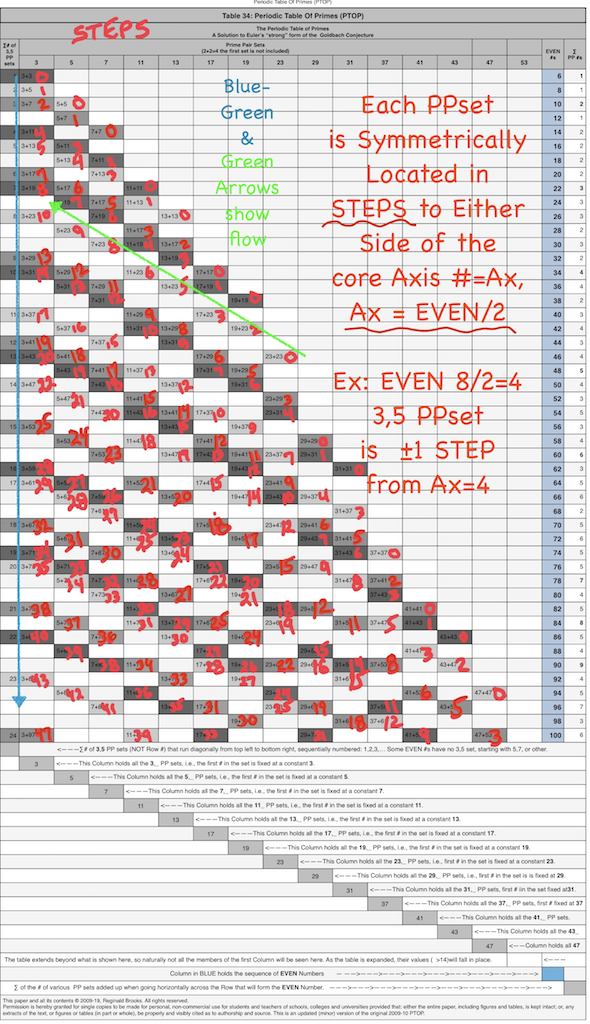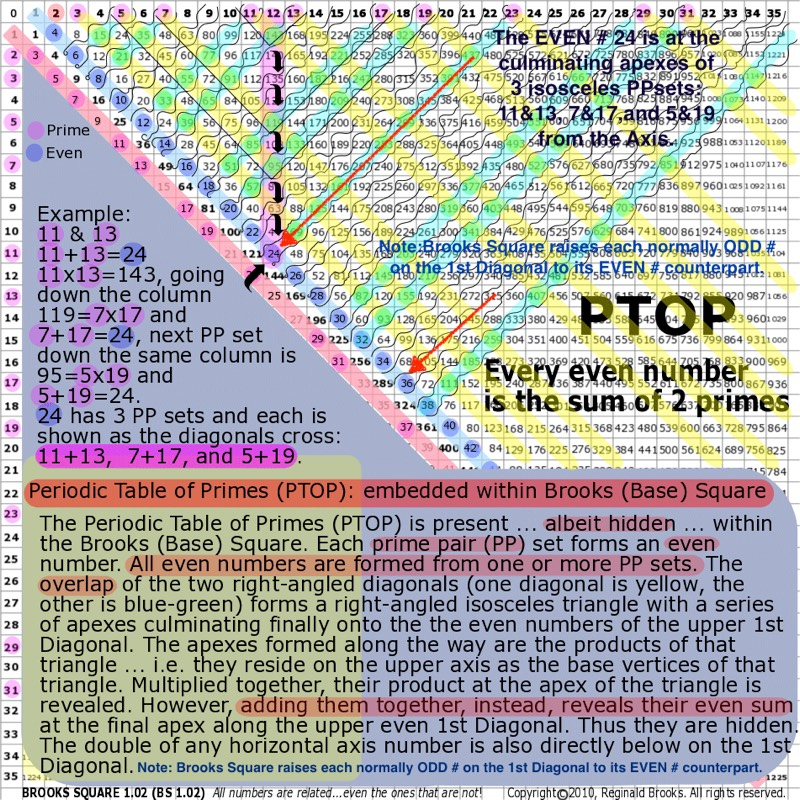Summary III. While the PTOP is "hidden" – i.e. not immediately obvious – on the BIM, with a slight tweak to the 1st Parallel Diagonal raising each ODD to an EVEN, the straight-line path back to the Axis points to the core Axis value of that EVEN. To either side, the members of the PPset(s) forming said EVEN, are symmetrically found as identical steps to either side. A line diagonally back from the PPset members forms at the intersection of straight-line path a 90° Right isosceles triangle. We have then, two forms of the fractal: the PS-Fractal that informs the spacing of the PPset TRAILS, and, the Fractal-Right-isosceles-triangle that presents each PPset on the BIM.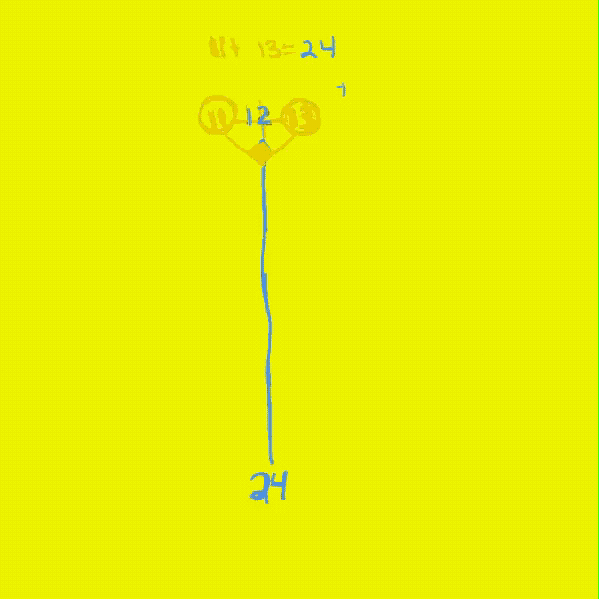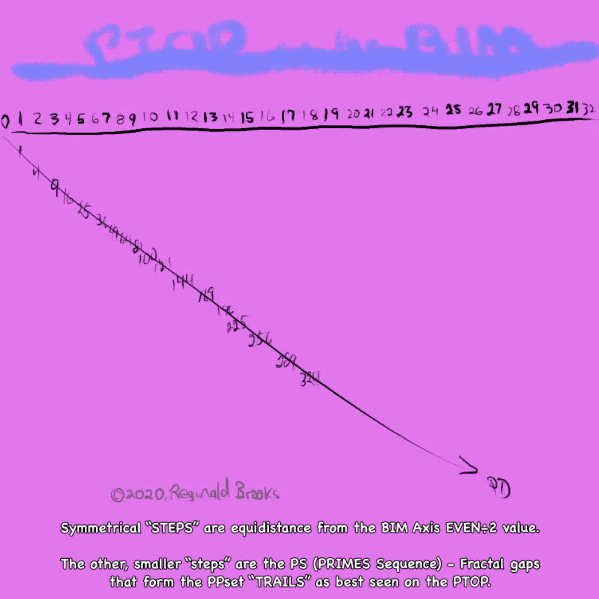Summary IV.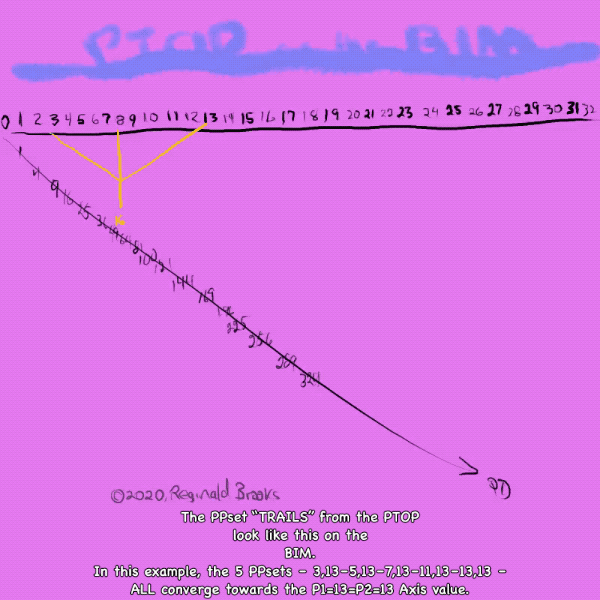Summary V.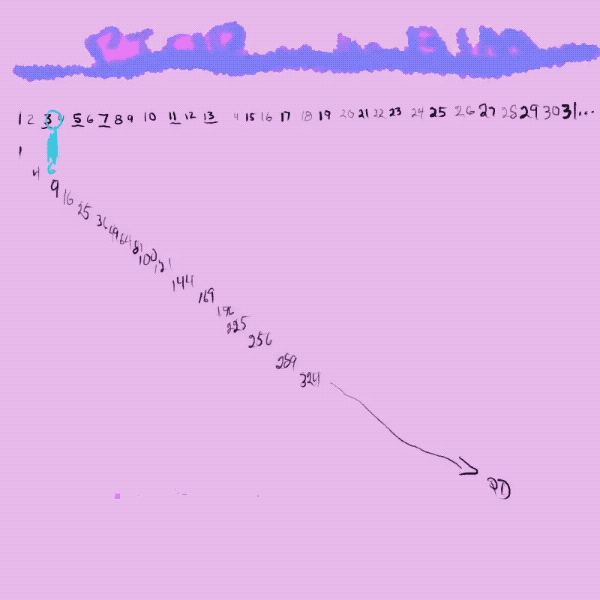Summary VI.

• Fig. 12- animated gif of video (below)
•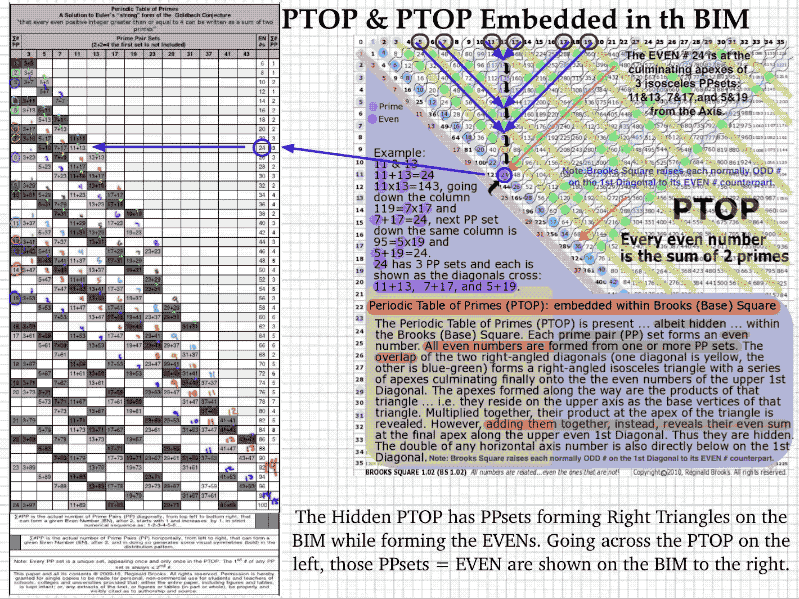• Video: PTOPrule170Annotated.mov
• (https://vimeo.com/372243091)

PTOP & Goldbach Conjecture from Reginald Brooks on Vimeo.

• Video: PTOP & Goldbach Conjecture: HOW to Make.mov
• (https://vimeo.com/382078605)

MathspeedST: TPISC Media Center

MathspeedST: eBook (free)

Apple Books

Artist Link in iTunes Apple Books Store: Reginald Brooks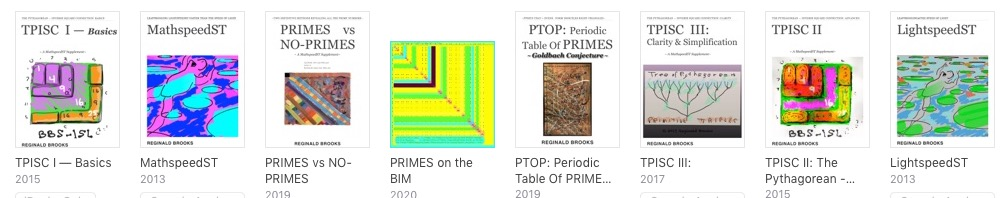BACK: ---> PRIMES Index on a separate White Paper

BACK: ---> Periodic Table Of PRIMES (PTOP) and the Goldbach Conjecture on a separate White Paper

BACK: ---> Periodic Table Of PRIMES (PTOP) - Goldbach Conjecture ebook on a separate White Paper

BACK: ---> Simple Path BIM to PRIMES on a separate White Paper

BACK: ---> PRIMES vs NO-PRIMES on a separate White Paper

BACK: ---> TPISC_IV: Details_BIM+PTs+PRIMES on a separate White Paper

BACK: ---> PRIME GAPS on a separate White Paper

Reginald Brooks

Brooks Design

Portland, OR

brooksdesign-ps.net

~~ ~~ ~~ ~~ ~~ ~~ ~~ ~~ ~~ ~~ ~~ ~~ ~~ ~~ ~~ ~~ ~~

KEYWORDS TAGS: PTOP, Periodic Table Of PRIMES, PRIMES vs NO-PRIMES, algebraic geometry, BIM, TPISC, The Pythagorean - Inverse Square Connections, Pythagorean Triangles, DNA, Zika virus, pentagon, decagon, double pentagon, composite axial DNA double-helix, Pythagorean Triples, primitive Pythagorean Triples, non-primitive Pythagorean Triples, Pythagorean Theorem, Pythagorus Theorem, The Dickson Method, BBS-ISL Matrix, Expanded Dickson Method, r-sets, s-set, t-sets, Pair-sets, geometric proofs, MathspeedST, leapfrogging LightspeedST FASTER than the speed of light, Brooks (Base) Square- Inverse Square Law (ISL), BBS-ISL Matrix grid, The Architecture Of SpaceTime (TAOST), The Conspicuous Absence Of Primes (TCAOP), A Fresh Piece Of Pi(e), AFPOP, Numbers of Inevitability, LightspeedST, Teachers, Educators and Students (TES), number theory, ubiquitous information, FASTER than the speed of light, primes, prime numbers, fractals, mathematics, Universe, cosmos, patterns in number, DSEQEC, Double-Slit Experiment-Quantum Entanglement Conjecture, CaCost, Creation and Conservation of SpaceTime.

Art Theory 101 / White Papers Index
 PIN: Pattern in Number...from primes to DNA. | PIN: Butterfly Primes...let the beauty seep in. | PIN: Butterfly Prime Directive...metamorphosis. | PIN: Butterfly Prime Determinant Number Array (DNA) ~conspicuous abstinence~. | GoDNA: the Geometry of DNA (axial view) revealed. | SCoDNA: the Structure and Chemistry of DNA (axial view). | The LUFE Matrix | The LUFE Matrix Supplement | The LUFE Matrix: Infinite Dimensions | The LUFE Matrix: E=mc2 | Dark Matter=Dark Energy | The History of the Universe in Scalar Graphics | The History of the Universe_update: The Big Void | Quantum Gravity ...by the book | The Conservation of SpaceTime | LUFE: The Layman's Unified Field Expose` | GoMAS: The Geometry of Music, Art and Structure ...linking science, art and esthetics. Part I | Brooks (Base) Square (BBS): The Architecture of Space-Time (TAOST) and The Conspicuous Absence of Primes (TCAOP) - a brief introduction to the series | more White Papers... net.art index | netart01: RealSurReal...aClone, 2001 | netart02: Hey!Ufunk'n with my DNA? | netart03: 9-11_remembered | netart04: Naughty Physics (a.k.a. The LUFE Matrix) | netart05: Your sFace or Mine? | netart06: Butterfly Primes | netart07: Music-Color-ISL | netart08: BBS-ISL matrix | netart09: BBS-interactive (I) | netart10: Sunspots and Solar Flares | netart11: Music-Color-ISL (II-III) | Art Theory 101: PIN, DNA, LUFE Matrix, GoMAS, BBS index | home Copyright 2019-20, Reginald Brooks, Brooks Design. All rights reserved. iTunes, iTunes Store, Apple Books, iBooks, iBooks Store, iBooks Author, Mac OS are registered® trademarks of Apple Inc. and their use on this webpage does not reflect endorsement by Apple Inc.
TOP